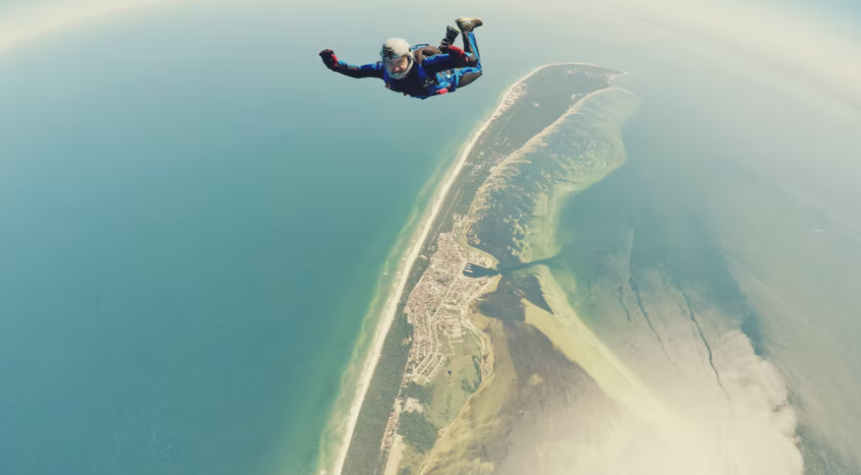## Terminal Velocity

Find the terminal velocity of a 70 kg skydiver falling in a spread eagle position if their 1.1 m^2 projected area has an associated drag coefficient of 1.5. Assume the density of air is 1.22 kg/m^3.Hint
The drag force on objects immersed in a large body of flowing fluid or objects moving through a stagnant fluid:
$$D=\frac{1}{2}\rho U^{2}C_{D}A$$$where $$C_D$$ is the drag coefficient, $$U$$ is the flowing fluid or moving object’s velocity, $$\rho$$ is the fluid density, and $$A$$ is the projected area of blunt objects with axes perpendicular to the flow. Hint 2 $$Force=mass \times acceleration$$$
The drag force on objects immersed in a large body of flowing fluid or objects moving through a stagnant fluid:
$$D_f=\frac{1}{2}\rho U^{2}C_{D}A$$$where $$C_D$$ is the drag coefficient, $$U$$ is the flowing fluid or moving object’s velocity, $$\rho$$ is the fluid density, and $$A$$ is the projected area of blunt objects with axes perpendicular to the flow. At terminal velocity, the net force is zero, meaning the drag force on the skydiver is equal to the force of gravity. Remember, $$Force=mass \times acceleration$$ , so $$F_{weight}=mg=D_f$$$
where $$m$$ is the mass and $$g$$ is the acceleration due to gravity.
Thus,
$$mg=\frac{1}{2}\rho U^{2}C_{D}A$$$Solving for terminal velocity: $$U=\sqrt{\frac{2mg}{\rho C_DA}}=\sqrt{\frac{2(70kg)(9.8m)(m^3)}{(s^2)(1.22kg)(1.5)(1.1m^2)}}$$$
$$=\sqrt{\frac{1372m^2}{2.013s^2}}=\sqrt{681.57m^2/s^2}=26.1\:m/s$$\$
26.1 m/s